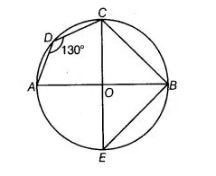# In figure, ∠ADC = 130° and chord`
Question:

In figure, ∠ADC = 130° and chord BC = chord BE. Find ∠CBE.Solution:

We have, ∠ADC = 130° and chord BC – chord BE. Suppose, we consider the points A, B, C and D form a cyclic quadrilateral.

Since, the sum of opposite angles of a cyclic quadrilateral ΔDCB is 180°.

=> 130°+ ∠OBC = 180°

=> ∠OBC = 180° -130° = 50°

In ΔBOC and ΔBOE,

BC = BE   [given equal chord]

OC =OE  [both are the radius of the circle]

and OB = OB [common side]

ΔBOC ≅ΔBOE

∠OBC = ∠OBE = 50° [by CPCT]

∠CBE = ∠CBO + ∠EBO = 50° + 50° = 100°